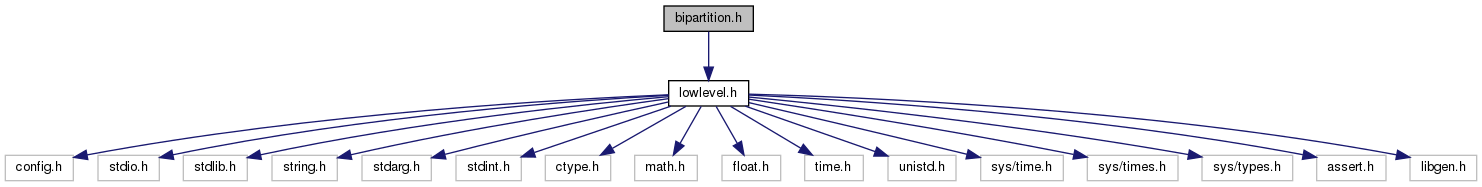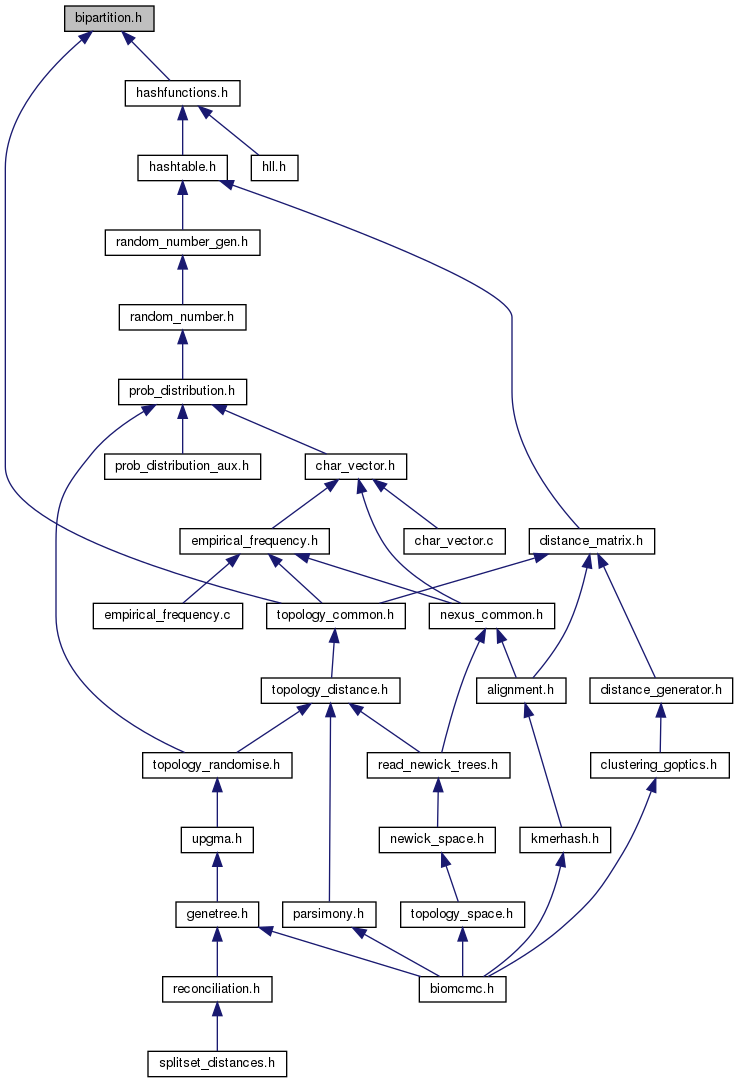biomcmc-lib  0.1 low level library for phylogenetic analysis
bipartition.h File Reference

Unary/binary operators on arbitrarily-sized bitstrings (strings of zeros and ones) like split bipartitions. More...

`#include "lowlevel.h"`
Include dependency graph for bipartition.h:This graph shows which files directly or indirectly include this file:Go to the source code of this file.

## Data Structures

struct  bipartition_struct
Bit-string representation of splits. More...

struct  bipsize_struct

## Typedefs

typedef struct bipartition_structbipartition

typedef struct bipsize_structbipsize

typedef bipartitiontripartition

## Functions

bipartition new_bipartition (int size)
create a new bipartition (bitstring) capable of storing an arbitrary number of bits and initialize it to zero More...

bipsize new_bipsize (int size)
Create a new bipsize, which controls some bipartition sizes.

bipartition new_bipartition_copy_from (const bipartition from)
Create a new bipartition (allocate memory) and initialize it from another bipartition.

bipartition new_bipartition_from_bipsize (bipsize n)
create new bipartition that will share bipsize – useful for bipartition vectors

void del_bipartition (bipartition bip)
free memory allocated by bipartition

void del_bipsize (bipsize n)
free memory allocated by bipsize

void bipsize_resize (bipsize n, int nbits)
update the valid number of bits and mask – e.g. when replacing subtrees by leaves in reduced trees

void bipartition_initialize (bipartition bip, int position)
set all bits to zero except the one at position-th bit

void bipartition_zero (bipartition bip)
set all bits to zero

void bipartition_set (bipartition bip, int position)
simply set the bit at "position" to one, irrespective of other bits

void bipartition_set_lowlevel (bipartition bip, int i, int j)

void bipartition_unset (bipartition bip, int position)
simply unset the bit at "position" (set to zero), irrespective of other bits

void bipartition_unset_lowlevel (bipartition bip, int i, int j)

void bipartition_copy (bipartition to, const bipartition from)
Copy contents from one bipartition to another.

void bipartition_OR (bipartition result, const bipartition b1, const bipartition b2, bool update_count)
Binary logical OR ("|") between b1 and b2, where update_count should be true if you need to know the resulting size (slow) or false if you don't care or if b1 and b2 are disjoint (no common elements)

void bipartition_AND (bipartition result, const bipartition b1, const bipartition b2, bool update_count)
Binary logical AND ("&") between b1 and b2, update_count should be set to false only if you really don't need to know the number of active bits (e.g. sorting, bipartition comparison)

void bipartition_ANDNOT (bipartition result, const bipartition b1, const bipartition b2, bool update_count)
Binary logical AND ("&") between b1 and ~b2 (NOT b2), that is, apply mask b1 on the inverse of b2.

void bipartition_XOR (bipartition result, const bipartition b1, const bipartition b2, bool update_count)
Binary logical eXclusive OR ("^") between b1 and b2, update_count should be set to false only if you really don't need to know the number of active bits (e.g. sorting, bipartition comparison)

void bipartition_XORNOT (bipartition result, const bipartition b1, const bipartition b2, bool update_count)
Binary logical eXclusive OR ("^") between b1 and complement of b2 (that is, NOT b2: b1 ^ ~b2). Used when finding best disagreement (that in this case erases the complement –other side – of agreement edge)

void bipartition_NOT (bipartition result, const bipartition bip)
Unary complement ("~") of bipartition. Use with caution, since there is no mask for unused padded bits.

void bipartition_count_n_ones (const bipartition bip)
Count the number of active bits (equal to one). Used by bipartition_AND() and bipartition_XOR() when update_count = true. Please use it parsimoniously since it is as slow as without bitstring representation.

void bipartition_to_int_vector (const bipartition b, int *id, int vecsize)
fill vector id[] with positions of set bits, up to vecsize bits set

bool bipartition_is_equal (const bipartition b1, const bipartition b2)
Compare equality of two bipartitions.

bool bipartition_is_equal_bothsides (const bipartition b1, const bipartition b2)
Compare if two bipartitions represent the same splits (or they are equal or one is the complement of the other)

int compare_bipartitions_increasing (const void *a1, const void *a2)
Bipartitions comparison, to be used by sort() since returns integer and uses (void)

int compare_bipartitions_decreasing (const void *a1, const void *a2)
Bipartitions comparison, to be used by sort() since returns integer and uses (void)

bool bipartition_is_larger (const bipartition b1, const bipartition b2)
Compare sizes of two bipartitions, by number of active bits with ties broken by actual bitstrings.

void bipartition_flip_to_smaller_set (bipartition bip)
invert ones and zeroes in loco when necessary to assure bipartition has more zeroes than ones

bool bipartition_is_bit_set (const bipartition bip, int position)
Check if position-th bit is equal to one or not.

bool bipartition_contains_bits (const bipartition b1, const bipartition b2)
Check if first bipartition contains all elements of second bipartition (b2 is a subset of b1)

void bipartition_print_to_stdout (const bipartition b1)
Print to screen a bit representation of the bipartition (with number of ones at the end)

void bipartition_replace_bit_in_vector (bipartition *bvec, int n_b, int to, int from, bool reduce)
replace bit info, copying 'from' one position 'to' another; bool "update" indicates if afterwards size will be reduced

void bipartition_resize_vector (bipartition *bvec, int n_b)
apply mask to last element (useful after manipulations) and count number of bits

tripartition new_tripartition (int nleaves)
tripartition of a node (a vector with 3 bipartitions, that should not be 'flipped' to smaller set, however)

void del_tripartition (tripartition trip)
free tripartition space (just 3 bipartitions)

void store_tripartition_from_bipartitions (tripartition tri, bipartition b1, bipartition b2)
from node, create tripartition from node->left and node->right (assuming bipartitions were not 'flipped' yet)

void sort_tripartition (tripartition tri)
sort order of bipartitions s.t. smallest is first

int align_tripartitions (tripartition tp1, tripartition tp2, hungarian h)
match bipartitions between two nodes and return optimal score (min disagreement)

bool tripartition_is_equal (tripartition tp1, tripartition tp2)
assuming tripartitions are ordered, check if nodes (represented by tripartitions) are the same

## Detailed Description

Unary/binary operators on arbitrarily-sized bitstrings (strings of zeros and ones) like split bipartitions.

## ◆ new_bipartition()

 bipartition new_bipartition ( int size )

create a new bipartition (bitstring) capable of storing an arbitrary number of bits and initialize it to zero

Parameters
 [in] size number of bits of desired bipartition
Returns
bipartition (opaquely a vector of long long ints)